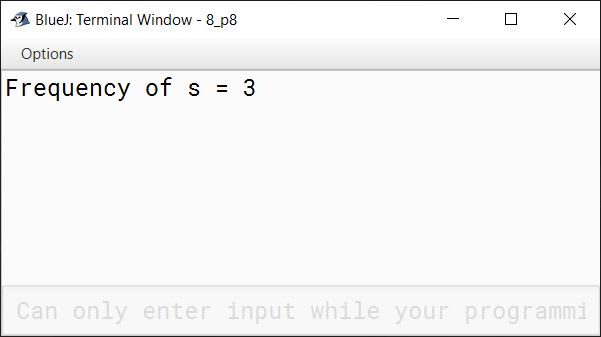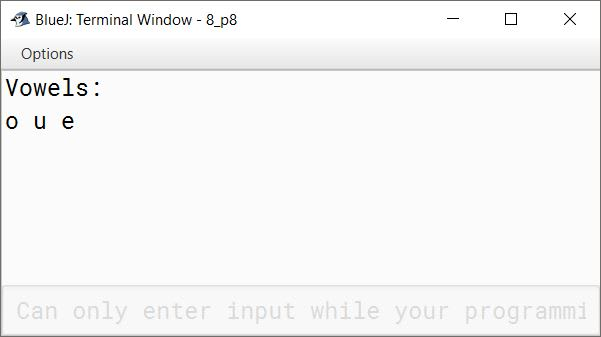# String Handling

## Objective Type Questions

#### Question 1

A String object cannot be modified after it is created. (T/F)

True

Reason — The string objects of Java are immutable i.e., once created, they cannot be changed. If any change occurs in a string object, then original string remains unchanged and a new string is created with the changed string.

#### Question 2

The length of a String object s1 can be obtained using the expression s1 length. (T/F)

False

Reason — The length of a String object s1 can be obtained using the expression s1.length() as length() method returns the length of the String object.

#### Question 3

Which of the following methods belong to the String class ?

1. length()
2. compareTo()
3. equals()
4. substring()
5. All of these
6. None of them

All of these

Reason — All of the given methods belong to the String class.

#### Question 4

Given the code

```String s1 = "yes" ;
String s2 = "yes" ;
String s3 = new String(s1) ;```

Which of the following would equate to False ?

1. s1 == s2
2. s3 == s1
3. s1.equals(s2)
4. s3.equals(s1)

s3 == s1

Reason — Each of the four comparisons are explained below:

1. The first comparison uses the `==` operator to compare `s1` and `s2`. `==` operator checks for reference equality, i.e., whether both variables refer to the same object in memory. Since `s1` and `s2` have the same value and were created using the same string literal, they will refer to the same object in memory. Therefore, this comparison returns `true`.
2. The second comparison uses the `==` operator to compare `s3` and `s1`. Since `s3` was created using the `new` keyword and is therefore a different object in memory than `s1`, this comparison returns `false`.
3. The third comparison uses the `equals` method to compare `s1` and `s2`. This method checks for value equality, i.e., whether both objects have the same value regardless of their memory location. Since `s1` and `s2` have the same value, this comparison returns `true`.
4. The fourth comparison uses the `equals` method to compare `s3` and `s1`. This method also checks for value equality, so it returns `true` because `s3` and `s1` have the same value, even though they are different objects in memory.

#### Question 5

Suppose that s1 and s2 are two strings. Which of the statements or expressions are incorrect ?

1. String s3 = s1 + s2;
2. String s3 = s1 - s2;
3. s1.compareTo(s2);
4. int m = s1.length( );

String = s1 - s2;

Reason`-` operator cannot be used with String objects, hence the statement `String s3 = s1 - s2;` is incorrect.

#### Question 6

Given the code :

`String s = new String("abc");`

Which of the following calls are invalid ?

1. s.trim( )
2. s.replace('a', 'A')
3. s.substring(3)
4. s.toUpperCase( )
5. s.setCharAt(1, 'A')

s.setCharAt(1, 'A')

Reason — setCharAt() is a method of StringBuffer class. It cannot be used with a String object, which is immutable.

#### Question 7

Which of these class is superclass of String and StringBuffer class?

1. java.util
2. java.lang
3. ArrayList
4. None of the mentioned

java.lang

Reason — java.lang is the superclass of String and StringBuffer class.

#### Question 8

Which of these methods of class String is used to obtain length of String object?

1. get( )
2. Sizeof( )
3. lengthof( )
4. length( )

length( )

Reason — length( ) returns the length of a given String object.

#### Question 9

Which of these methods of class String is used to extract a single character from a String object?

1. CHARAT( )
2. chatat( )
3. charAt( )
4. ChatAt( )

charAt( )

Reason — charAt( ) returns the character at the given index in a String object.

#### Question 10

Which of these constructors is used to create an empty String object?

1. String( )
2. String(void)
3. String(0)
4. None of the mentioned

String( )

Reason — String( ) constructor is used to create an empty String object as it does not specify any String value.

#### Question 11

Which of these is an incorrect statement?

1. String objects are immutable, they cannot be changed.
2. When you assign a new value to a String object, Java internally creates another String object.
3. StringBuffer is a mutable class that can store sequence of characters.
4. String objects are actually arrays of characters.

String objects are actually arrays of characters.

Reason — String are classes in Java. Array of characters is one of the data members of String class.

## Assignment Questions

#### Question 1

What are String and StringBuffer classes of Java?

In Java, we can work with characters by using String and StringBuffer class.

String class — The instances of String class can hold unchanging string, which once initialized cannot be modified. For example,

`String s1 = new String("Hello");`

StringBuffer class — The instances of StringBuffer class can hold mutable strings, that can be changed or modified. For example,

`StringBuffer sb1 = new StringBuffer("Hello");`

#### Question 2

How are Strings different from StringBuffers ?

The string objects of Java are immutable i.e., once created, they cannot be changed. If any change occurs in a string object, then original string remains unchanged and a new string is created with the changed string.

On the other hand, StringBuffer objects are mutable. Thus, these objects can be manipulated and modified as desired.

#### Question 3

How can you convert a numeric value enclosed in a string format ?

We can convert a numeric value enclosed in a string format into a numeric value by using valueOf() method.

For example, the given statement will return the numeric value of the String argument `"15"`.

`int num = Integer.valueOf("15");`

#### Question 4

Write the return type of the following library functions :

1. isLetterOrDigit(char)
2. replace(char, char)

1. boolean
2. String

#### Question 5

State the data type and values of a and b after the following segment is executed.

```String s1 = "Computer", s2 = "Applications";
a = (s1.compareTo(s2));
b = (s1.equals(s2));```

Data type of a is int and value is 2. Data type of b is boolean and value is false.

##### Explanation

compareTo() method compares two strings lexicographically and returns the difference between the ASCII values of the first differing characters in the strings. Thus, `a` will be of `int` data type and store the value `2` as the difference between ASCII values of 'A' and 'C' is 2.

equals() method compares two String objects and returns `true` if the strings are same, else it returns `false`. Thus, `b` will be of `boolean` data type and result in `false` as both the strings are not equal.

#### Question 6

What do the following functions return for :

```String x = "hello";
String y = "world";```

(i) System.out.println(x + y);

(ii) System.out.println(x.length( ));

(iii) System.out.println(x.charAt(3));

(iv) System.out.println(x.equals(y));

(i) System.out.println(x + y);

##### Output
``````helloworld
``````
##### Explanation

`+` operator concatenates String objects `x` and `y`.

(ii) System.out.println(x.length( ));

##### Output
``````5
``````
##### Explanation

length() returns the length of the String object. Since `x` has 5 characters, the length of the string is 5.

(iii) System.out.println(x.charAt(3));

##### Output
``````l
``````
##### Explanation

chatAt() returns the character at the given index. Index starts from 0 and continues till length - 1. Thus, the character at index `3` is `l`.

(iv) System.out.println(x.equals(y));

##### Output
``````false
``````
##### Explanation

equals() method checks for value equality, i.e., whether both objects have the same value regardless of their memory location. As values of `x` and `y` are not the same, so the method returns `false`.

#### Question 7

What will the following code output ?

```String s = "malayalam" ;
System.out.println(s.indexOf('m'));
System.out.println(s.lastIndexOf('m'));```

##### Output
``````0
8
``````
##### Explanation

indexOf() returns the index of the first occurrence of the specified character within the current String object. The first occurrence of 'm' is at index `0`, thus 0 is printed first.

lastIndexOf() returns the index of the last occurrence of the specified character within the String object. The last occurrence of 'm' is at index `8`, thus 8 is printed next.

#### Question 8

If:

```String x = "Computer";
String y = "Applications";```

What do the following functions return?

(i) System.out.println(x.substring(1,5));

(ii) System.out.println(x.indexOf(x.charAt(4)));

(iii) System.out.println(y + x.substring(5));

(iv) System.out.println(x.equals(y));

(i) System.out.println(x.substring(1,5));

##### Output
``````ompu
``````
##### Explanation

`x.substring(1,5)` will return a substring of x starting at index 1 till index 4 (i.e. 5 - 1 = 4).

(ii) System.out.println(x.indexOf(x.charAt(4)));

##### Output
``````4
``````
##### Explanation

charAt() method returns the character at a given index in a String. Thus, `x.charAt(4)` will return the character at the 4th index i.e., `u`. indexOf() method returns the index of the first occurrence of a given character in a string. Thus, it returns the index of `u`, which is `4`.

(iii) System.out.println(y + x.substring(5));

##### Output
``````Applicationster
``````
##### Explanation

`x.substring(5)` will return the substring of `x` starting at index 5 till the end of the string. It is "ter". This is added to the end of string `y` and printed to the console as output.

(iv) System.out.println(x.equals(y));

##### Output
``````false
``````
##### Explanation

`equals()` method checks for value equality, i.e., whether both objects have the same value regardless of their memory location. As the values of both `x` and `y` are different so it returns `false`.

#### Question 9

State the method that:

(i) converts a string to a primitive float data type.

(ii) determines if the specified character is an uppercase character.

(i) Float.parseFloat()

(ii) Character.isUpperCase()

#### Question 10

What will be the output of the following code snippet when combined with suitable declarations and run?

```StringBuffer city = new StringBuffer("Madras");
StringBuffer string = new StringBuffer( );
string.append(new String(city));
string.insert(0, "Central ");
String.out.println(string);```

The given snippet will generate an error in the statement — `String.out.println(string);` as the correct statement for printing is — `System.out.println(string);`. After this correction is done, the output will be as follows:

##### Output
``````Central Madras
``````
##### Explanation
StatementRemark
`StringBuffer city = new StringBuffer("Madras");`It creates a StringBuffer object`city` which stores "Madras".
`StringBuffer string = new StringBuffer( );`It creates an empty StringBuffer object `string`.
`string.append(new String(city));`It adds the value of `city` i.e. "Madras" at the end of the StringBuffer object `string`. string = " Madras".
`string.insert(0, "Central ");`It modifies StringBuffer object `string` and adds "Central" at 0 index or beginning. Now, string = "Central Madras".
`System.out.println(string);`It prints "Central Madras".

#### Question 11

What will be the output for the following program segment?

```String s = new String ("abc");
System.out.println(s.toUpperCase( ));```

##### Output
``````ABC
``````
##### Explanation

String `s` stores "abc".

toUpperCase( ) method converts all of the characters in the String to upper case. Thus, "abc" is converted to "ABC" and printed on the output screen.

#### Question 12

Give the output of the following program :

```class MainString
{
public static void main(String[ ] args)
{
StringBuffer s = new StringBuffer("String");
if((s.length( ) > 5) &&
(s.append("Buffer").equals("X")))
;	// empty statement
System.out.println(s);
}
}```

##### Output
``````StringBuffer
``````
##### Explanation

The expression `s.length( ) > 5` will result in `true` because the length of String `s` is `6` which is `> 5`.

In the expression `s.append("Buffer".equals("X"))`, first the value of String `s` will be modified from `String` to `StringBuffer` and then it will be compared to `"X"`. The result will be `false` as both the values are not equal.

Now, the condition of if statement can be solved as follows:

``````if((s.length( ) > 5) && (s.append("Buffer").equals("X")))
if(true && false)
if(false)
``````

Thus, execution will move to the next statement following the if block — `System.out.println(s);` which will print `StringBuffer` on the screen.

#### Question 13

Write a program to do the following :

(a) To output the question "Who is the inventor of Java" ?

(c) To print out "Good" and then stop, if the answer is correct.

(d) To output the message "try again", if the answer is wrong.

(e) To display the correct answer when the answer is wrong even at the third attempt and stop.

```import java.util.Scanner;

public class KboatQuiz
{
public static void main(String args[])
{
Scanner in = new Scanner(System.in);

String q = "Who is the inventor of JAVA?";
String a = "James Gosling";
int i;

System.out.println(q);

for(i = 1; i <= 3; i++)
{
String ans = in.nextLine();
ans.trim();
if(ans.equalsIgnoreCase(a))
{
System.out.println("Good");
break;
}
else if(i < 3)
{
System.out.println("Try Again");
}
}

if(i == 4)
{
}
}
}```
##### Output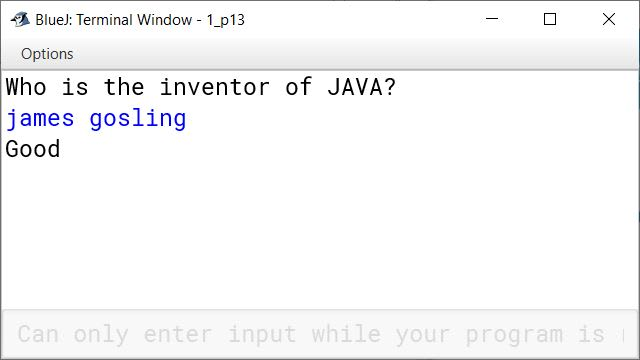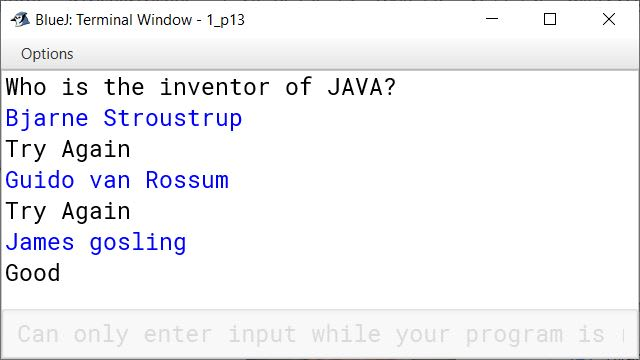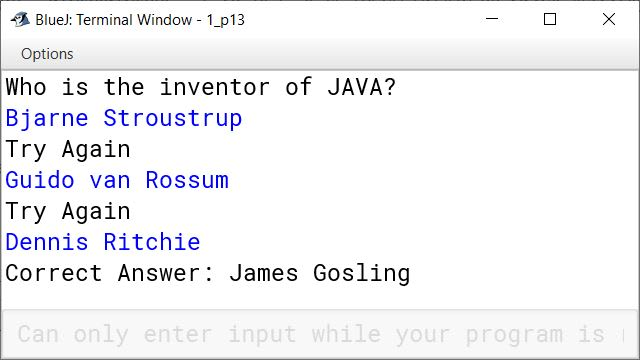#### Question 14

Write a program to extract a portion of a character string and print the extracted string. Assume that m characters are extracted, starting with the nth character.

```import java.util.Scanner;

public class KboatExtractSubstring
{
public static void main(String args[])
{
Scanner in = new Scanner(System.in);
System.out.print("Enter string : ");
String s = in.nextLine();
int len = s.length();
System.out.print("Enter start index : ");
int n = in.nextInt();

if(n < 0 || n >= len)
{
System.out.println("Invalid index");
System.exit(1);
}

System.out.print("Enter no. of characters to extract : ");
int m = in.nextInt();

int substrlen = n + m;
if( m <= 0 || substrlen > len)
{
System.out.println("Invalid no. of characters");
System.exit(1);
}

String substr = s.substring(n, substrlen);
System.out.println("Substring = " + substr);
}
}```
##### Output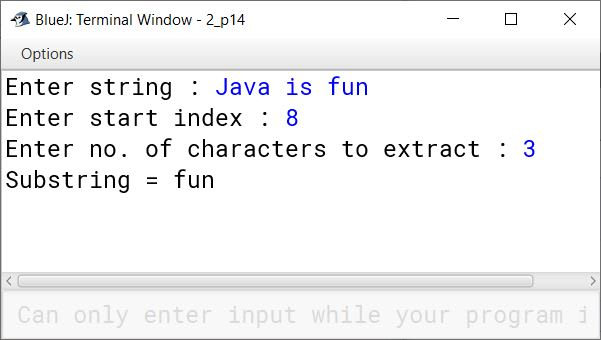#### Question 15

Write a program, which will get text string and count all occurrences of a particular word.

```import java.util.Scanner;

public class KboatWordFrequency
{
public static void main(String args[]) {
Scanner in = new Scanner(System.in);
System.out.println("Enter a sentence:");
String str = in.nextLine();
System.out.println("Enter a word:");
String ipWord = in.nextLine();
str += " ";
String word = "";
int count = 0;
int len = str.length();

for (int i = 0; i < len; i++) {
if (str.charAt(i) == ' ') {

if (word.equalsIgnoreCase(ipWord))
count++ ;

word = "";
}
else {
word += str.charAt(i);
}
}

if (count > 0) {
System.out.println(ipWord + " is present " + count + " times.");
}
else {
System.out.println(ipWord + " is not present in sentence.");
}

}
}```
##### Output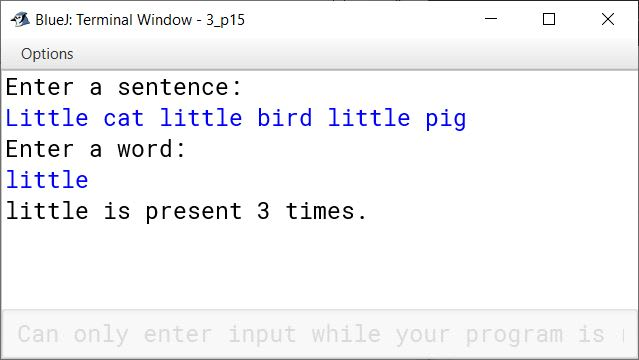#### Question 16

Write a program to accept a string. Convert the string to uppercase. Count and output the number of double letter sequences that exist in the string.

Sample Input : "SHE WAS FEEDING THE LITTLE RABBIT WITH AN APPLE"

Sample Output : 4

```import java.util.Scanner;

public class KboatLetterSeq
{
public static void main(String args[]) {
Scanner in = new Scanner(System.in);
System.out.print("Enter string: ");
String s = in.nextLine();
String str = s.toUpperCase();
int count = 0;
int len = str.length();

for (int i = 0; i < len - 1; i++) {
if (str.charAt(i) == str.charAt(i + 1))
count++;
}

System.out.println("Double Letter Sequence Count = " + count);

}
}```
##### Output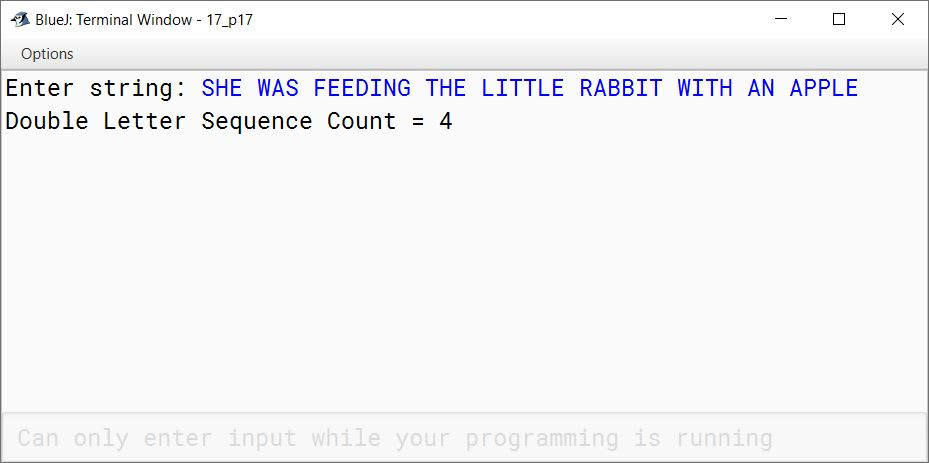#### Question 17

Design a class to overload a function check( ) as follows :

(i) void check(String str, char ch) - to find and print the frequency of a character in a string.
Example:
Input :
str = "success" ch= 's'

Output :
number of s present is = 3

(ii) void check (String s1) - to display only vowels from string s1, after converting it to lower case.
Example:
Input:
sl = "computer"
Output : o u e

```public class KboatOverload
{
void check (String str , char ch ) {
int count = 0;
int len = str.length();
for (int i = 0; i < str.length(); i++) {
char c = str.charAt(i);
if (ch == c) {
count++;
}
}
System.out.println("Frequency of " + ch + " = " + count);
}

void check(String s1) {
String s2 = s1.toLowerCase();
int len = s2.length();
System.out.println("Vowels:");
for (int i = 0; i < len; i++) {
char ch = s2.charAt(i);
if (ch == 'a' ||
ch == 'e' ||
ch == 'i' ||
ch == 'o' ||
ch == 'u')
System.out.print(ch + " ");
}
}
}```
##### Output